Question
How to prove G（n）=n+1 in this algorithm？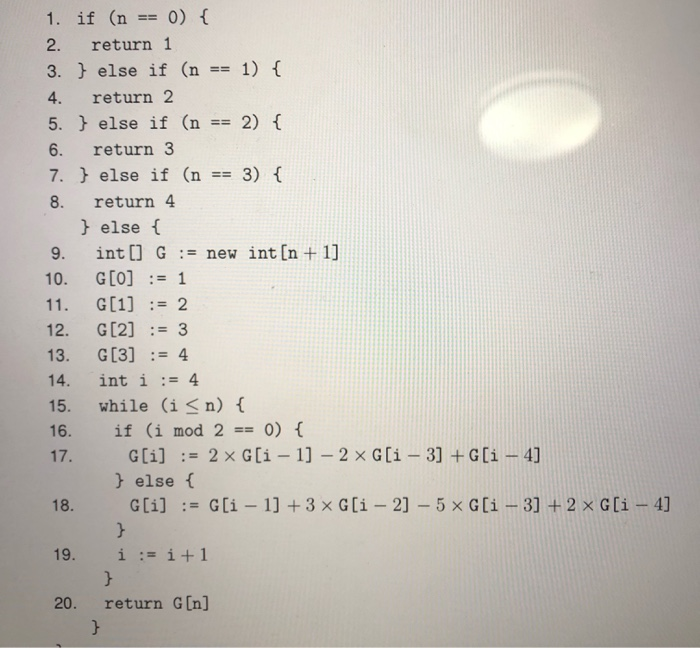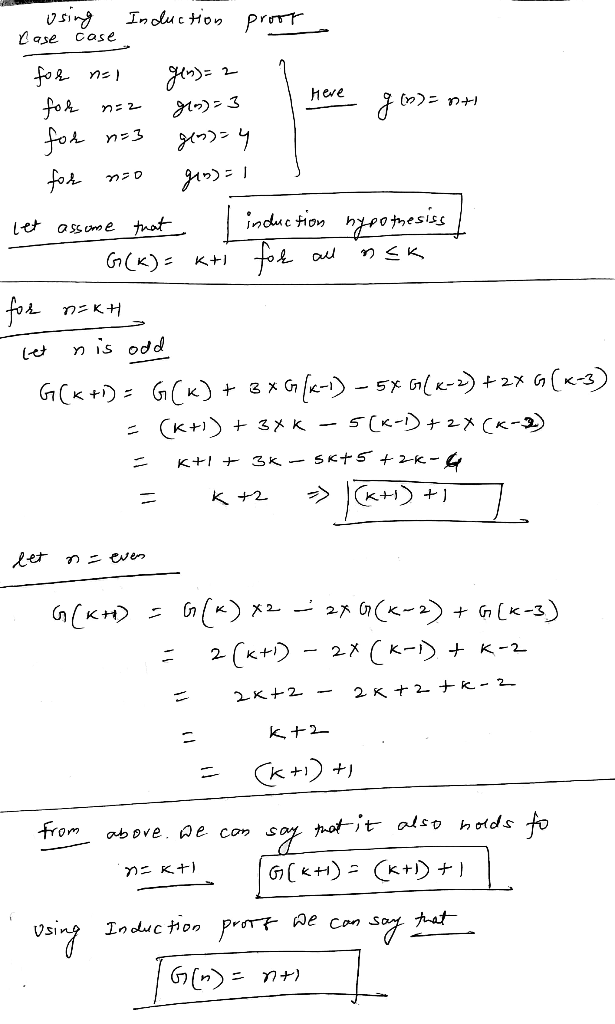#### Earn Coins

Coins can be redeemed for fabulous gifts.

Similar Homework Help Questions
• ### For questions 10-12, refer to the following iterative code computes values for the table t. 1 pub...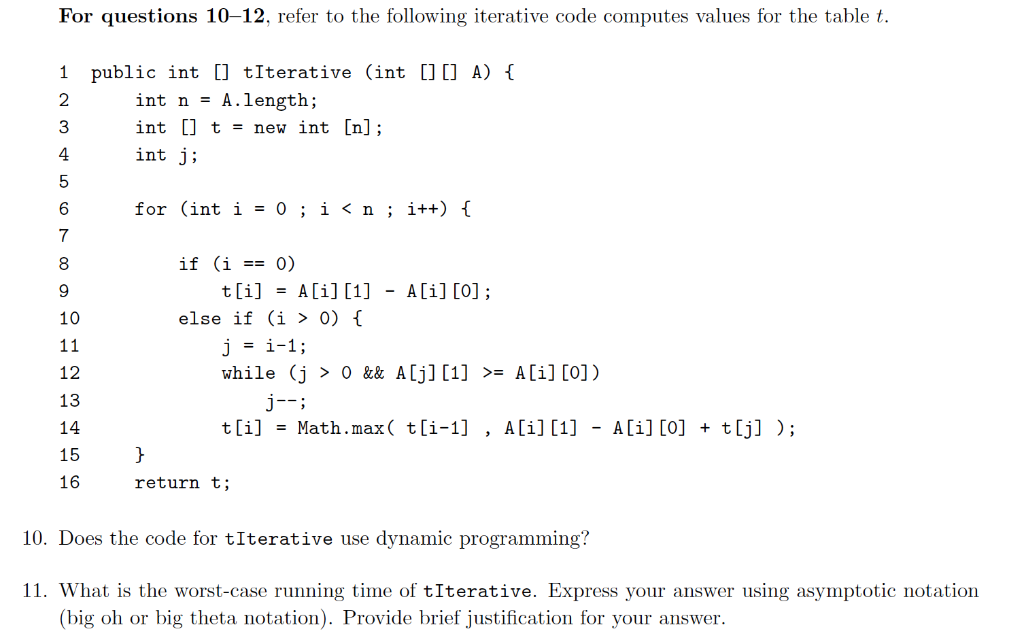For questions 10-12, refer to the following iterative code computes values for the table t. 1 public int [] tIterative (int [C A)1 2 3 4 int n - A.length; int [] t = new int [n]; int j; for (inti-0; i 0) while (j > 0 && A[j]  A[i] [O]) 12 13 14 15 16 t[i]Math.max( t[i-1] , A[i][i] - A[i] [o] + t[j] ); return t; 10. Does the code for tIterative use dynamic programming? 11. What...

• ### b) Consider the following code. public static int f(int n) if (n == 1) return 0;...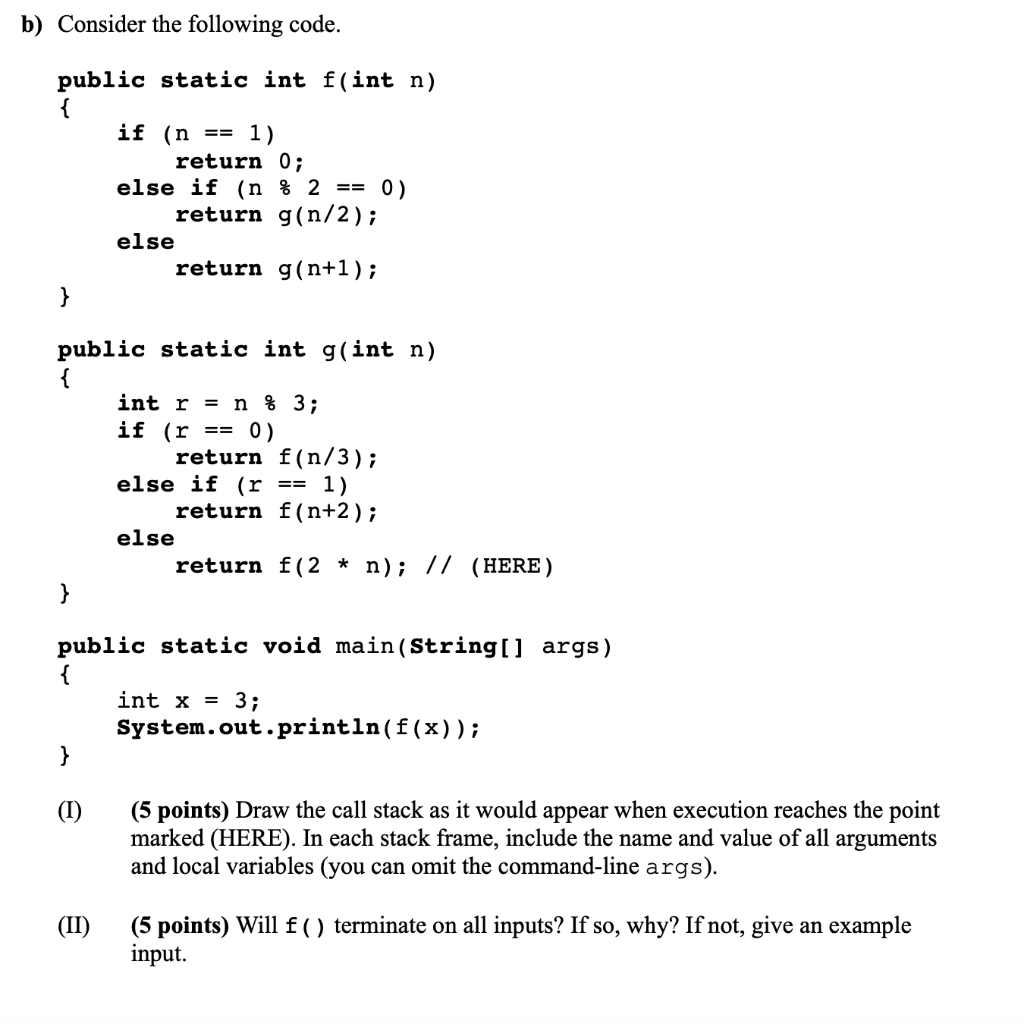b) Consider the following code. public static int f(int n) if (n == 1) return 0; else if (n % 2 == 0). return g(n/2); else return g(n+1); public static int g(int n) int r = n % 3; if (r == 0) return f(n/3); else if (r == 1) return f(n+2); else return f(2 * n); // (HERE) public static void main(String[] args) { int x = 3; System.out.println(f(x)); (1) (5 points) Draw the call stack as it would...

• ### f(t) -S -8 -7 -3 --1 3 5 1 2 13 4 7 8 9 12...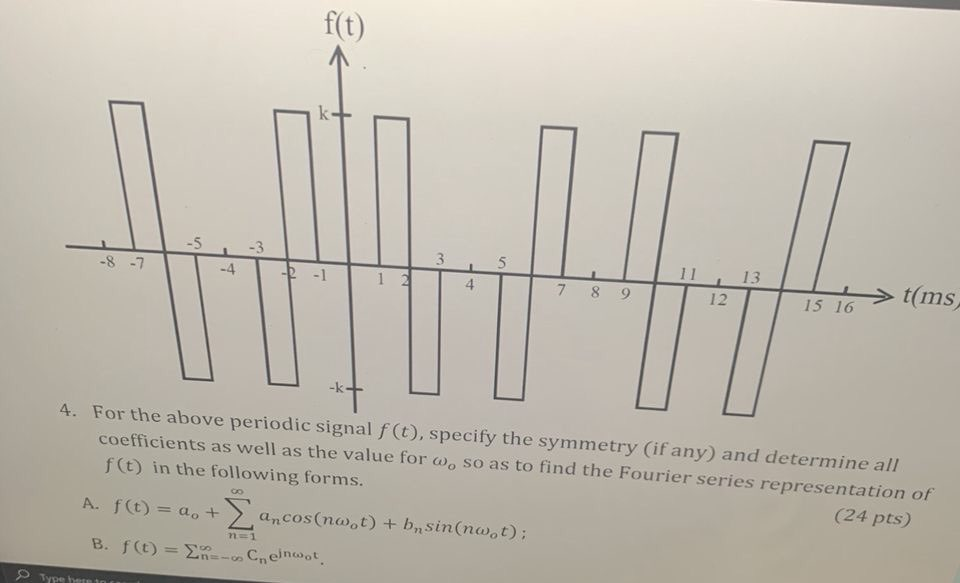f(t) -S -8 -7 -3 --1 3 5 1 2 13 4 7 8 9 12 t(ms 15 16 4. For the above periodic signal f(t), specify the symmetry (if any) and determine all coefficients as well as the value for w, so as to find the Fourier series representation of f(t) in the following forms. (24 pts) GO A. f(t) = a + ancos(not) + b sin(nw.t): B. f(t) = R-Cneinwor. n=1 Type A

• ### 10 1 2 3 4 5 6 7 8 9 HHHHHHHHH \$10 \$10 \$10 \$10 \$0...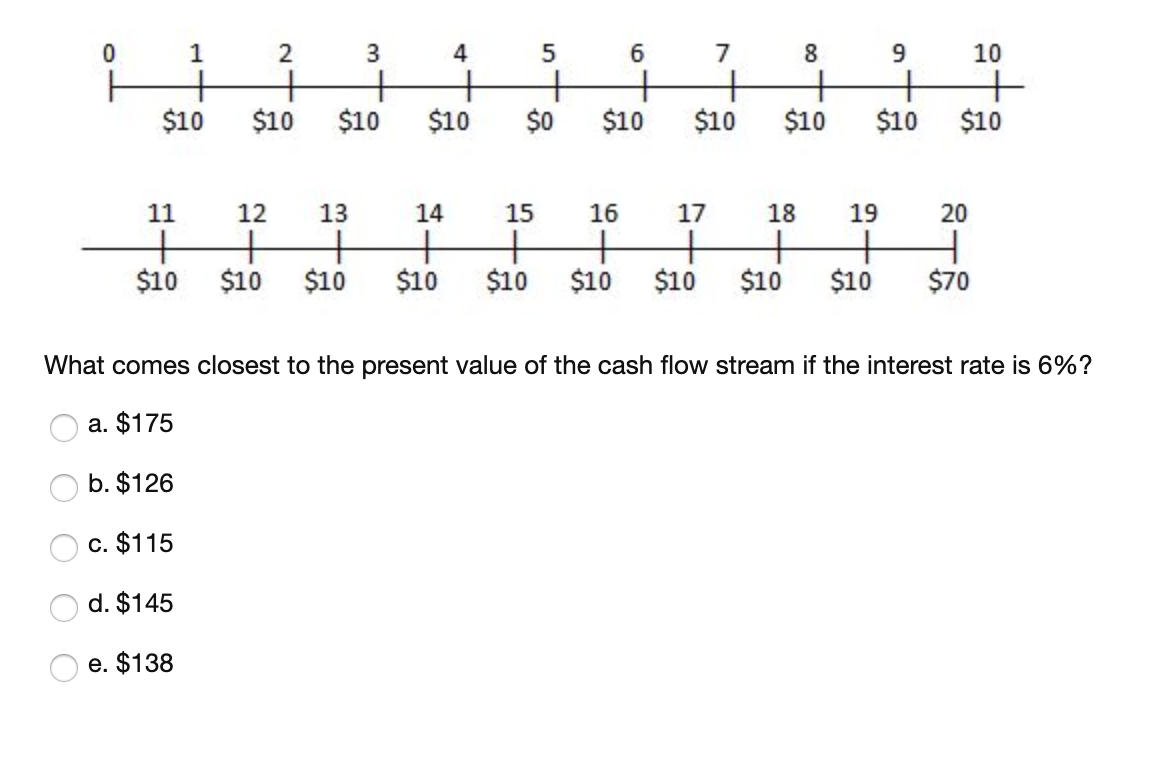10 1 2 3 4 5 6 7 8 9 HHHHHHHHH \$10 \$10 \$10 \$10 \$0 \$10 \$10 \$10 \$10 \$10 11 12 13 14 15 16 17 18 19 20 \$10 \$10 \$10 \$10 \$10 \$10 \$10 \$10 \$10 \$70 What comes closest to the present value of the cash flow stream if the interest rate is 6%? a. \$175 O b. \$126 O c. \$115 O d. \$145 o e. \$138

• ### Question 18 CLO3 Analyze the following code and answer the questions that follow def F(n): If n <= 1: return n else: return F(n-1)+F(n-2) for i in range (n) print (F(i)) Result: 0 1 1 2 3 5 8...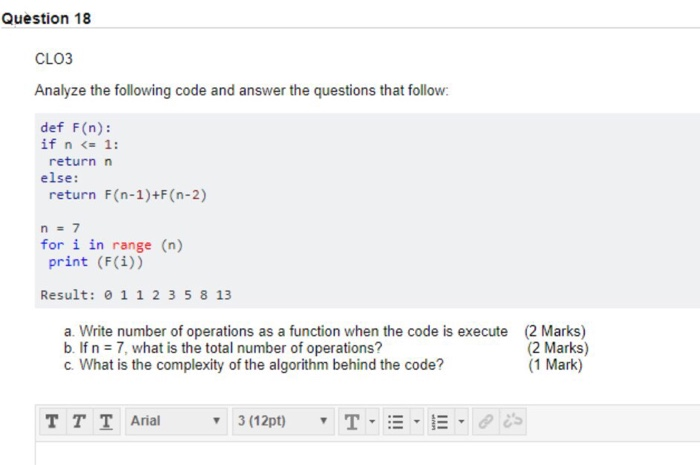Question 18 CLO3 Analyze the following code and answer the questions that follow def F(n): If n <= 1: return n else: return F(n-1)+F(n-2) for i in range (n) print (F(i)) Result: 0 1 1 2 3 5 8 13 a. Write number of operations as a function when the code is execute b If n 7, what is the total number of operations? c. What is the complexity of the algorithm behind the code? (2 Marks) (2 Marks) (1...

• ### Compute the sign of the following permutations: (a) (1, 4, 3, 6, 7)(5, 8, 9, 10). (b) σ ∈ Sn, i 7...

Compute the sign of the following permutations: (a) (1, 4, 3, 6, 7)(5, 8, 9, 10). (b) σ ∈ Sn, i 7→ n + 1 − i. (c) Show that this initial configuration of the 15 puzzle is not solvable 3 1 15 13 2 8 5 9 12 7 11 4 14 6 10 − (d) Suppose that (i, j) ∈ Sn is a transposition and that i < j. Find an expression (in terms of i and j...

• ### 1 #include <stdio.h> 2 3 11 Remember this written by the 4 // "new guy down...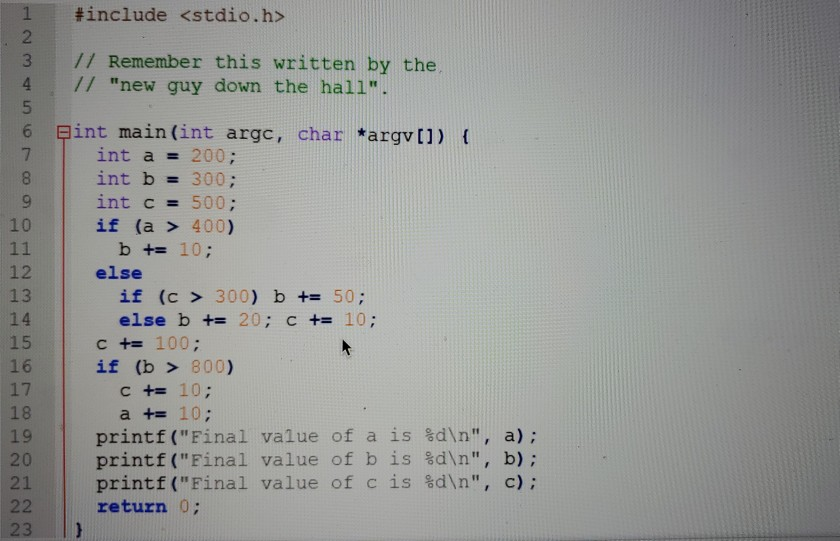1 #include <stdio.h> 2 3 11 Remember this written by the 4 // "new guy down the hall". 5 6 pint main(int argc, char *argv[]) { 7 int a = 200; 8 int b = 300; 9 int c = 500; 10 if (a > 400) 11 b += 10; 12 else 13 if (c > 300) b += 50; 14 else b += 20; C += 10; 15 C += 100; 16 if (b > 800) 17 C +=...

• ### 1) How many times is the fib method invoked for fb(5)7 a. 14 b. 15 c....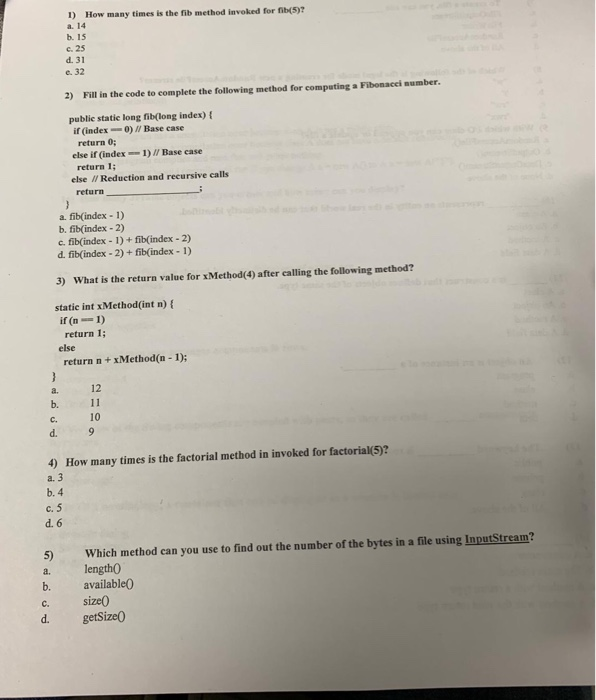1) How many times is the fib method invoked for fb(5)7 a. 14 b. 15 c. 25 d. 31 e. 32 2) Fill in the code to complete the following method for computing Fibonacei aumber publie statie long fib(long index) t if (index--0) Base case return 0; return 1 return else if (index1)/ Base case else I/ Reduction and recursive calls a. fib(index 1) b. fib(index-2) c. fib(index-1)+ fib(index -2) d. fib(index - 2) + fib(index -1) 3) What is...

• ### 3. Recursive Program (6 points) Consider the following recursive function for n 1: Algorithm 1 int...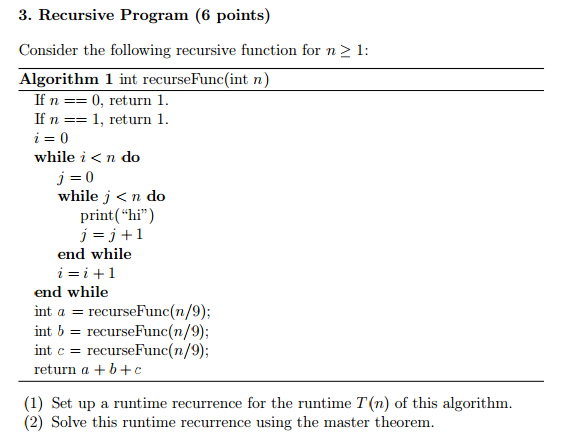3. Recursive Program (6 points) Consider the following recursive function for n 1: Algorithm 1 int recurseFunc(int n) If n 0, return 1. If n 1, return 1 while i< n do while j <n do print("hi") j 1 end while i i 1 end while int a recurse Func(n/9); int b recurse Func (n/9) int c recurse Func (n/9) return a b c (1) Set up a runtime recurrence for the runtime T n) of this algorithm. (2) Solve...

• ### QUESTION 8 What is the worst-case complexity of line 7 of function bar? A. O(1) B....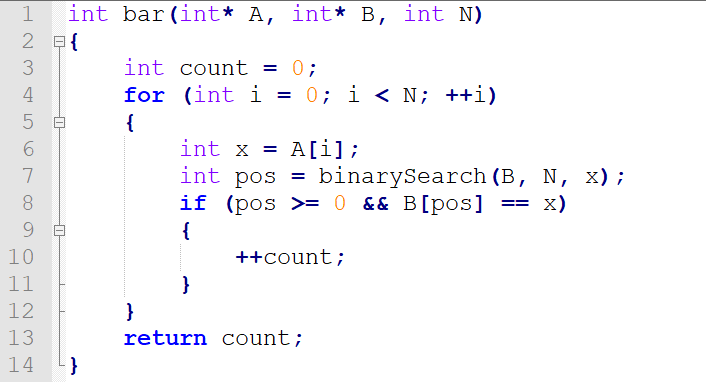QUESTION 8 What is the worst-case complexity of line 7 of function bar? A. O(1) B. O(N) C. O(i) D. O(log N) E. O(sqrt N) F. O(A[i]) G. O(N sqrt N) H. O(N log N) I. O(N^2) J. O(i^2) K. None of the above QUESTION 9 What is the worst-case complexity of lines 6-11 of function bar? A. O(1) B. O(N) C. O(i) D. O(log N) E. O(sqrt N) F. O(A[i]) G. O(N sqrt N) H. O(N log N) I....